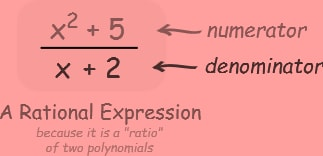Register for Maths, Science, English, Reasoning Olympiad Exams Register here | Book Free Demo for Live Olympiad Classes here | Check Olympiad Exam Dates here | Buy Practice Papers for IMO, IOM, HEO, IOEL etc here | Login here to participate in all India free mock test on every Saturday

# Rational ExpressionAn expression that is the ratio of two polynomials. It is "Rational" because one is divided by the other, like a ratio. Note: the polynomial we divide by cannot be zero.

70%